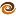# EmptyCrate

#### About Me | Contact Me | Training |ChaiScript.com | YouTube Channel

I felt like this topic deserved one more article. (See part 1 and part 2 for the background.)

I felt like this topic deserved one more article. (See part 1 and part 2 for the background.) The question was posed, “what if you make the list of integers dynamically generated?”

The question was posed, “what if you make the list of integers dynamically generated?”

So, I came up with this little generator iterator adaptor. It allows you to take any generator function and treat it like a forward iterator:

``````#include <iostream>
#include <algorithm>
template<typename Gen, typename Val, int Size>
class generator_itr
{
private:
Gen m_g;
Val m_cur;
int m_iteration;

public:
generator_itr end()
{
generator_itr i(*this);
i.m_iteration = Size + 1;
return i;
}

generator_itr(Gen t_g)
: m_g(t_g), m_cur(t_g()), m_iteration(0)
{
}

generator_itr(const generator_itr &t_itr)
: m_g(t_itr.m_g), m_cur(t_itr.m_cur), m_iteration(t_itr.m_iteration)
{
}

generator_itr &operator++() //prefix
{
m_cur = m_g();
++m_iteration;
return *this;
}

generator_itr operator++(int) //postfix
{
generator_itr t = *this;
m_cur = m_g();
++m_iteration;
return t;
}

const Val &operator*()
{
return m_cur;
}

const Val * const operator->()
{
return &m_cur;
}

bool operator==(const generator_itr &r)
{
return m_iteration == r.m_iteration;
}

bool operator!=(const generator_itr &r)
{
return m_iteration != r.m_iteration;
}
};

struct Sum
{
uint64_t m_sum;

Sum()
: m_sum(0)
{
}

void operator()(uint32_t i)
{
m_sum += i;
}
};

struct increment_generator
{
int m_value;

increment_generator(int i)
: m_value(i)
{
}

int operator()()
{
return m_value++;
}
};

int main()
{
//Create the generator iterator adaptor, for 65,000,000 entries
generator_itr<increment_generator, uint32_t, 65000000> itr(increment_generator(0));
//Use the generator iterator to sum 0..64999999
std::cout << std::for_each(itr, itr.end(), Sum()).m_sum << std::endl;
}
``````

How does this version compare to others? Over 1000 iterations (which is not what the above example shows), the performance is very similar to that of the versions from parts 1 and 2.

For just summing the integers once, it is much faster than the version that generates and stores the list.

What this does gain, however, is a huge boost in memory performance. Say, for instance, you wanted to sum a list of 65,000,000 (what the above does show) and it is relatively inexpensive to generate your list (like a linearly incremented list is) you can see performance differences like this:

To sum the list once:

• dynamically generated list: 0.161s
• statically generated list: 33.860s

Which is a 99.6% speed increase, or 210 times faster! Why the huge performance disparity? In my case I am using an Ubuntu VirtualBox set up, so I know that the 260MB of ram required to sum 65,000,000 32bit integers pushes my system into swapping to the disk to store and access that much memory. Disk is much much slower than RAM.

My Videos

Related